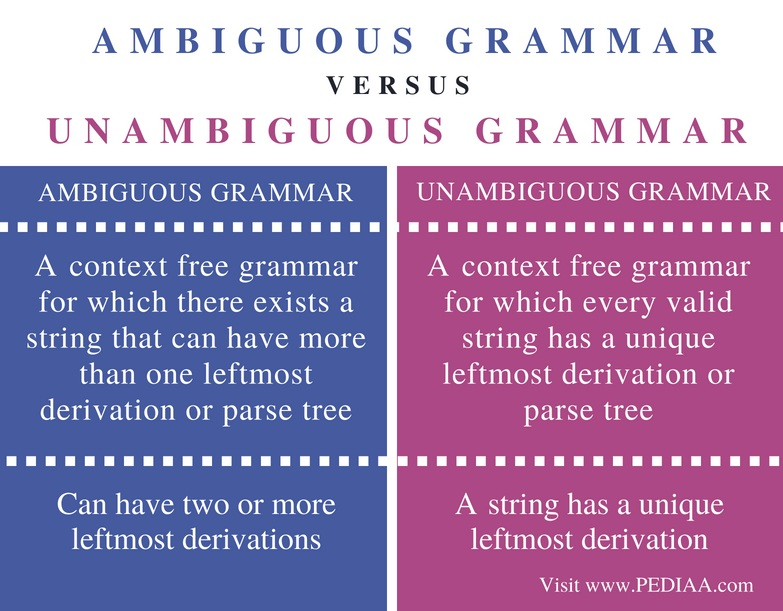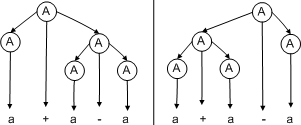# Difference Between Ambiguous and Unambiguous Grammar

The main difference between ambiguous and unambiguous grammar is that the ambiguous grammar is a context free grammar for which there exists a string that can have more than one leftmost derivation while an unambiguous grammar is a context free grammar for which every valid string has a unique leftmost derivation.

Grammar refers to the syntactical rules in natural languages. In 1956, computer scientists introduced a mathematical model of grammar for writing computer language. If it is possible to derive all the strings of a language using a certain grammar, then it is said that the language is generated from that grammar. Context free grammar is one type of grammar. This grammar generates context free language. The context free grammar can be ambiguous or unambiguous. For a particular string, if there are two or more derivations, that grammar is said to be ambiguous. For a particular string, if there is an only unique leftmost derivation, that grammar is said to be unambiguous grammar.

### Key Areas Covered

1. What is Ambiguous Grammar
– Definition, Example
2. What is Unambiguous Grammar
– Definition, Example
3. Difference Between Ambiguous and Unambiguous Grammar
– Comparison of Key Differences

### Key Terms

Ambiguous Grammar, Unambiguous Grammar## What is Ambiguous Grammar

A grammar is said to be ambiguous if there exist two or more derivations for a string.Figure 1: Ambiguous Grammar

Assume that there is a grammar defined as follows.

G= ({S}, {a+b, +, *}, P, S}. The production rules are as follows. S -> S+S | S*S | a | b. Assume that it is required to generate the String a+ a*b.

Consider, S -> S+S

Substituting ‘a’ for left most S will give the following.

S-> a +S

Substituting S*S for S is as follows.

S-> a + S*S

Substituting ‘a’ for the left most S will give the below output.

S -> a+ a*S

Substituting ‘b’ for the S will give the following output.

S -> a + a * b

This is the required string to generate.

When using the other production rule, it will give

S -> S* S

Apply S+S to the left most S will give the following.

S -> S+S * S

Substitute ‘a’ for left most S,

S -> a + S*S

Substituting ‘a’ for the left most S,

S -> a + a * S

Substituting ‘b’ for S will give the following output.

S -> a + a*b

Again, it generated the required string. Therefore, there is more than one derivation to generate the string. Therefore, it is an ambiguous grammar.

## What is Unambiguous Grammar

In an ambiguous grammar, a certain string has a unique leftmost derivation. Refer the following production rules.

S -> L | a, L -> LS | S

Consider the S -> L rule. Substitute LS instead of L.

S -> LS

Substitute S, for first L.

S -> S S

Substituting ‘a’ for the leftmost S will give the below output.

S -> a S

Substituting ‘a’ for S will give the following.

S -> a a

Therefore, a string has a unique leftmost derivation. So, it is an unambiguous grammar.

## Difference Between Ambiguous and Unambiguous Grammar

### Definition

An ambiguous grammar is a context free grammar for which there exists a string that can have more than one leftmost derivation or parse trees. Unambiguous grammar is a context free grammar for which every valid string has a unique leftmost derivation or parse tree.

### Number of Leftmost Derivations

In ambiguous grammar, a string can have two or more leftmost derivations but, in unambiguous grammar, a string has a unique leftmost derivation.

### Conclusion

Context free grammar can be ambiguous or unambiguous. The difference between ambiguous and unambiguous grammar is that the ambiguous grammar is a context free grammar for which there exists a string that can have more than one leftmost derivation while an unambiguous grammar is a context free grammar for which every valid string has a unique leftmost derivation.

##### Reference:

1. “Ambiguous Grammar.” Wikipedia, Wikimedia Foundation, 17 July 2018, Available here.
2. “Compiler Design | Ambiguous Grammar.” GeeksforGeeks, 10 Feb. 2018, Available here.
3. “Ambiguous Grammar”, Neso Academy, 29 Mar. 2017, Available here.

##### Image Courtesy:

1. “Leftmostderivations jaredwf” By Jaredwf at English Wikipedia – Transferred from en.wikipedia to Commons by EdwardHades (Public Domain) via Commons Wikimedia